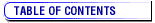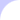Last update:07-Jul-2004Arch Hellen Med, 21(2), March-April 2004, 172-178 APPLIED MEDICAL RESEARCH The logistic regression model in diagnostic research T. KATOSTARAS Faculty of Nursing, University of Athens, Athens, Greece

The logistic regression model is the most important statistical method used, mainly in etiological research, but also in diagnostic and prognostic research. In diagnostic research with the use of this model, the probability of a disease is estimated according to the presence of other characteristics, called determinants. The logistic regression model is applied in studies on cohort and open populations. Between the members of the population, the ratio of the percentage of the population displaying a specific disease to the percentage of the population without the disease (ratio of the complementary probabilities) is called the odds percentage of the existence of the disease. Given that the determinant is present, the ratio of the odds of the existence probability of a rare disease to the odds of the absence probability of the disease, the odds ratio (OR) is taken approximately as the estimation of the relative risk (RR) of the appearance of the disease, for the given factor. This approximating equation justifies the use of the OR in order to correlate the presentation of a disease with the presence of a determinant. The logistic regression model is a non-linear statistical model used to estimate the probability of a specific characteristic (disease) in the members of a population when specific determinants are present. With the use of the estimated coefficients of the model, the OR is estimated and the reliability limits are calculated for each determinant, which are the corresponding estimations of the RR for each determinant. There are various ways of controlling whether the logistic regression model that has been estimated can be used in a specific case. One way is to study its capability for proper classification, with calculation of the different percentages of classification. When the values of the variables of each unit are substituted in the estimated model, the probability of the disease can be calculated. Using this probability, the individuals examined are classified into those estimated to have and those to have not the disease. If the percentages of accurate classification are high, the model is suitable for use in the diagnostic procedure.

Key words: Diagnostic research, Logistic regression, Medical research, Multiple logistic model, Multivariate analysis.